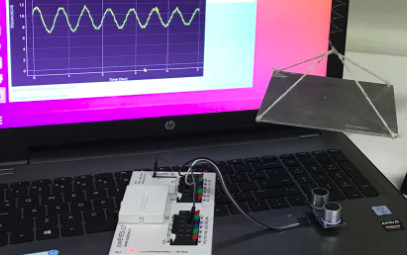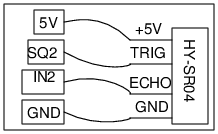# Measuring Distance using Sound Echo

## Distance measurement using HY-SR04 Piezo sensorBrief Summary
HY-SR04 is a widely available ultrasound ranging module. It consists of two 40 kHz Piezo crystals, where one acts as a transmitter and other as a receiver, and some electronics circuitry is inbuilt to measure the time interval between a burst of sound transmitted and the echo received. This module can measure distance with around 3mm Accuracy, and can be applied to study mass-spring systems, pendulums, and other similar mechanical systems.

## Introduction

The four pins on the SR04 module are the power supply(5V), ground, trigger(from SQ2) and Echo(Measured by IN2). This module can be connected to ExpEYES-17 as shown in the schematic, to measure distance between the module and the reflecting surface, with an error less than 3mm.

This can be used to design several experiments in mechanics where moving objects need to be studied without touching them.## The Mass and Spring problem

When a mass suspended on a spring is made to oscillate, the period of oscillation is given by

$T = 2 * \pi * \sqrt\frac{m}{k}$

where m is the mass and k is the spring constant.

We can measure the period of oscillation by measuring the distance to the moving mass as a function of time. The distance versus time graph is fitted with a sine function to calculate the frequency. The photograph below shows a metal plate suspended on a spring and the SR04 facing it from the bottom. The distance to the oscillating plate is measured for 5 seconds and the data is fitted to a sine function.## Simple Pendulum

The period of oscillations of a simple pendulum also can be similarly measured using SR04 connected to ExpEYES. The figure shows a pendulum with a rectangular bob, but one can use a spherical bob with a piece of paper (to provide a flat reflecting surface) pasted to it. The measured frequency is 1.05 Hz for a pendulum having a length of 22cm. One can calculate the value of acceleration due to gravity from this data using the well known theoretical formula.